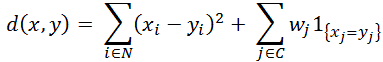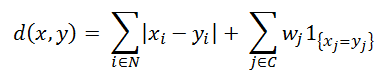# 1.1 - 8.10 - KModes (ML Engine) - Teradata Vantage

## Teradata Vantage™ - Machine Learning Engine Analytic Function Reference

Product
Release Number
1.1
8.10
Release Date
October 2019
Content Type
Programming Reference
Publication ID
B700-4003-079K
Language
English (United States)

KModes is an extension of KMeans that supports categorical data. KModes models are fit similarly to KMeans models. The core algorithm is an expectation-maximization algorithm that finds a locally optimal solution.

These are the main steps to fitting the model:

1. Initialization

Select a set of K initial cluster centers. You can create this set with the RandomSample (ML Engine) function, which samples rows from an input table using the kmeans++ and kmeans|| algorithms. These initialization algorithms create initial cluster centers that are more likely to lead to better local optima.

2. E step

A mapper assigns each point in the input table to one of the K clusters, and stores the sums of the numerical attributes and counts of the categorical attributes.

3. M step

A reducer aggregates the the statistics created for each worker in the E step and creates new cluster centers. For numerical attributes, the new center is the mean of the value of the attribute for the points assigned to the cluster. For categorical attributes, the new center is the mode of the attribute value for the points assigned to the cluster.

The algorithm runs for either a set number of iterations or until the change in movement of the cluster centers drops below a user-specified threshold.

When assigning points to a cluster, a hybrid distance function that combines a numeric distance and a categorical distance is required. The default distance between two data points in a KModes model is the squared Euclidean distance:where N denotes the indexes of numerical attributes, C denotes the indexes of categorical attributes, and wj denotes the weight to assign to a category.

The Manhattan distance can also be used: